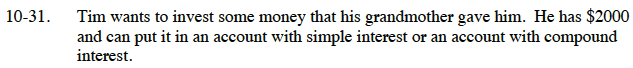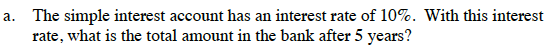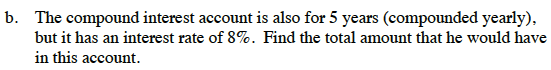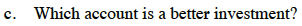### Home > CC3 > Chapter Ch10 > Lesson 10.1.2 > Problem10-31

10-31.
1. Tim wants to invest some money that his grandmother gave him. He has $2000 and can put it in an account with simple interest or an account with compound interest. Homework Help ✎ 1. The simple interest account has an interest rate of 10%. With this interest rate, what is the total amount in the bank after 5 years? 2. The compound interest account is also for 5 years (compounded yearly), but it has an interest rate of 8%. Find the total amount that he would have in this account. 3. Which account is a better investment?Add the interest he earns to his initial principal to find the total amount he would have after 5 years. I = ($2000)(.1)(5 years)
I = $1000 Total =$2000 + $1000 =$3000A = 2000(1 + 0.8)5

(1.08)5 = 1.469

A = $2000 (1.469) =$2938.66Which account has more money after 5 years?